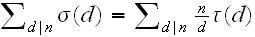# Number theory function

This is not a homework , i got this question from the internet.

Prove:#### Attachments

We have:
$$\sum_{d|n} \frac{n}{d}\tau(d) = \sum_{d | n} d \tau(n/d)$$
There is a bijective correspondence between divisors of n/d and multiples of d that divide n. Thus $\tau(n/d)$ equals the number of integers k such that d | k and k | n. Now $\sigma(d)$ equals the sum of all divisors of d so on the left-hand side we have $\tau(n/d)$ terms of d which shows the equality. To establish this through a somewhat more formal argument define:
$$S = \{(d,k) \,:\, d |k, k | n\}$$
For a finite subset T of $\mathbb{Z}^2$ define:
$$w(T) = \sum_{(d,k) \in T} d$$
This has the nice property that if $A,B \subseteq \mathbb{Z}^2$, then,
$$w(A \cup B) = w(A) + w(B) - w(A \cap B)$$
We wish to show that both our sides of the equality equal w(S). To do this we decompose S in two ways. First define
$$S_d = \{(d,k) \,:\, d|k, k |n\}$$
Then $|S_d|=\tau(n/d)$ so $w(S_d) = d\tau(n/d)$. We can then show:
$$w(S) = \sum_{d|n} w(S_d) = \sum_{d|n} d\tau(n/d)$$
This deal with the right-hand side. On the other hand let
$$T_k = \{(d,k) \,:\, d|k, k |n\}$$
Then $w(T_k) = \sum_{d|k} d = \sigma(k)$, which shows:
$$w(S) = \sum_{d|n} w(T_d) = \sum_{d|n} \sigma(d)$$

Hi rasmhop !
I am new to number theoretic function , i don't understand what do you mean by bijective correspondence and your sets notation.
I was given a hint to start of by starting to prove for the case of $$p^{k}$$ instead of n , such that p is a prime number. could you explain in a slightly elementary level.

Ok. I'm not sure at exactly what level you want the explanation, but let me try a somewhat more elementary approach using your hint. I use some manipulations of sums, but I don't know how familiar you are with this stuff. If there is something you're not clear on just ask. Anyway the general idea is to first prove the case for prime powers, then prove the both the left-hand side and right-hand side are multiplicative.

Let us consider the case $n=p^k$ for some prime p and positive integer k. We have
$$\sigma(p^c) = 1+p+p^2+\cdots+p^c$$
so for our left-hand side we have:
\begin{align*} \sum_{d | p^k} \sigma(d) &= \sum_{i=0}^k \sigma(p^i) \\ &= \sum_{i=0}^k \sum_{j=0}^i p^j \end{align*}
It's clear that $p^j$ occurs in this sum once every time $i \geq j$ so the same number of times as the number of elements of $\{j,j+1,\ldots,k\}$ which is k-j+1 so:
$$\sum_{d | p^k} \sigma(d) = \sum_{j=0}^k (k-j+1)p^j$$
On the other hand we have $\tau(p^i) = i+1$ which gives:
\begin{align*} \sum_{d | n} \frac{n}{d}\tau(d) &= \sum_{i=0}^k \frac{p^k}{p^i} \tau(p^i) \\ &= \sum_{i=0}^k p^{k-i}(i+1)\\ &= \sum_{i=0}^k p^{i}(k-i+1) \\ &= \sum_{d | p^k} \sigma(d) \end{align*}
which shows the case when n is a prime power.

Now we need to use this knowledge to gain an understanding of the general case. Let us assume a and b are coprime and that the case has been proven for both a and b (for instance if a and b are different prime powers), and let us show that the result also holds for ab. Then the result will follow by induction. We know that $\tau$ and $\sigma$ are multiplicative so $\tau(ab) = \tau(a)\tau(b)$ and $\sigma(ab)=\sigma(a)\sigma(b)$. If $d_1 | a$ and $d_2 | b$, then $d_1d_2|ab$. Also if $d | ab$, then there exists unique positive integers $d_1,d_2$ such that $d_1 | a$ and $d_2 | b$ (since a and b are coprime). Thus we have:
$$\sum_{d |ab} \frac{ab}{d}\tau(d) = \sum_{d_1|a, d_2|b} \frac{ab}{d_1d_2}\tau(d_1d_2)$$
$$\sum_{d |ab}\sigma(d) = \sum_{d_1|a, d_2|b} \sigma(d_1d_2)$$
Now we use this to prove that both the left-hand side and the right hand side are multiplicative:
\begin{align*} \sum_{d |ab}\sigma(d) &= \sum_{d_1|a, d_2|b} \sigma(d_1d_2) \\ &= \sum_{d_1|a, d_2|b} \sigma(d_1)\sigma(d_2) \\ &= \sum_{d_1|a}\sum_{d_2 | b}\sigma(d_1)\sigma(d_2) \\ &= \sum_{d_1|a}\sigma(d_1)\sum_{d_2 | b}\sigma(d_2) \\ &= \left(\sum_{d_1|a}\sigma(d_1)\right)\left(\sum_{d_2 | b}\sigma(d_2)\right) \end{align*}
And:
\begin{align*} \sum_{d|ab}\frac{ab}{d}\tau(d) &= \sum_{d_1|a,d_2|b}\frac{ab}{d_1 d_2}\tau(d_1 d_2) \\ &= \sum_{d_1|a,d_2|b}\frac{a}{d_1}\tau(d_1)\frac{b}{d_2}\tau(d_2) \\ &= \sum_{d_1|a}\left(\frac{a}{d_1}\tau(d_1)\sum_{d_2 | b}\frac{b}{d_2}\tau(d_2)\right) \\ &= \left(\sum_{d_1|a}\frac{a}{d_1}\tau(d_1)\right)\left(\sum_{d_2 | b}\frac{b}{d_2}\tau(d_2)\right) \end{align*}
Now since we assumed the result was true for a and b we have:
$$\sum_{d|ab}\frac{ab}{d}\tau(d) = \left(\sum_{d_1|a}\frac{a}{d_1}\tau(d_1)\right)\left(\sum_{d_2 | b}\frac{b}{d_2}\tau(d_2)\right) = \left(\sum_{d_1|a}\sigma(d_1)\right)\left(\sum_{d_2 | b}\sigma(d_2)\right) = \sum_{d |ab}\sigma(d)$$